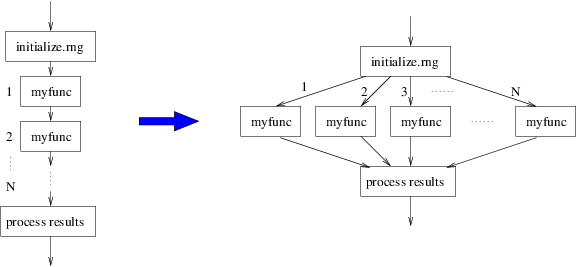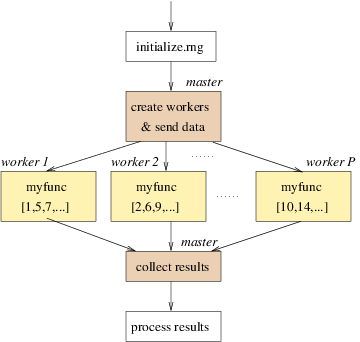# Required Packages

``````install.packages(c("foreach", "doParallel", "doRNG",
"reshape2", "wpp2017"),
dependencies = TRUE)``````

# Structure of Statistical Simulations

Many statistical simulations have the following structure:

``````initialize.rng(...)
for (iteration in 1:N) {
result[iteration] <- myfunc(...)
}
process(result,...)``````

If calls of `myfunc` are independent of one another, we can transform the simulation as follows:• A cluster consists of a master and a set of workers, usually one worker per physical node.
• Each worker is responsible for one or more calls of `myfunc`.
• Master is responsible for sending workers everything they need and for collecting results.• Usually, number of workers P is much smaller than the number of tasks N.

There are many packages in R that work in this fashion. One of the first packages, snow (Simple Network of Workstations) has been recently re-implemented as an R core package called parallel.

# Package parallel

• The parallel package contains implementations of two packages:
• snow (Luke Tierney at al)
• multicore (Simon Urbanek)
• The snow part works for homogeneous as well as heterogeneous clusters on any OS.
• The multicore part works for Mac/Unix/Linux OS (not Windows) and is designed for multi CPU/core single computers with shared memory. It’s main functions are `mclapply`, `mcmapply` and `mcMap`.
• In this tutorial we will focus on the snow part of parallel.

## Setup

• Load the package and check how many cores you have:

``````library(parallel)
P <- detectCores(logical = FALSE) # physical cores
P``````

## Start and Stop a Cluster

• Start and stop a pool of workers with one worker per core:

``````cl <- makeCluster(P)
cl
typeof(cl)
length(cl)
cl[]
cl[[P]]
typeof(cl[[P]])
names(cl[[P]])
stopCluster(cl)
# cl[] # gives an error``````

### Types of Clusters

• Socket communication (default):

``````cl <- makeCluster(P, type = "PSOCK")
stopCluster(cl)``````
• Available for all OS platforms.
• Fork: `type = "FORK"`
• Workers are complete copies of the master process.
• Not available for Windows.
• MPI: `type = "MPI"`
• Requires the Rmpi package (and MPI) to be installed.
• NetWorkSpaces: `type = "NWS"`
• Requires the nws package (from Revolution Computing) to be installed.

## Evaluating a Function on the Cluster

• Start a cluster that will be used to solve multiple tasks:

``cl <- makeCluster(P)``
• Let’s get each worker to generate as many normally distributed random numbers as its position in the list:

``clusterApply(cl, 1:P, fun = rnorm)``

The second argument is a sequence where each element gets passed to the corresponding worker, namely as the first argument to the function `fun`. In this example, the first worker got the number 1, second 2 etc. which is passed as the first argument to the `rnorm` function. Thus, the node `cl[]` for example evaluates `rnorm(4, mean = 0, sd = 1)`.

• Pass additional arguments to `fun`:

``````clusterApply(cl, 1:P, fun = rnorm,
mean = 10, sd = 2)``````
• Evaluate a function more times than the number of workers: Generate 20 sets of 100,000 random numbers from N(mean=5, sd=1) and return the average of each set:

``````res <- clusterApply(cl, rep(100000, 20),
fun = function(x) mean(rnorm(x, mean = 5)))
length(res)